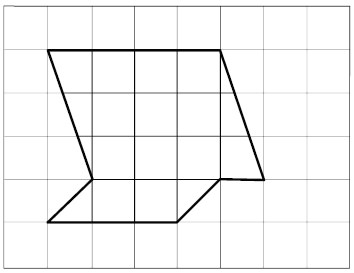Home | | Geography 11th std | Measurement of Area

# Measurement of Area

The measurement of a geographical area can be carried out on a map.

Measurement of Area

The measurement of a geographical area can be carried out on a map. There are number of methods used to measure area on a map. One of the simplest methods is by means of similar squares.Square method: The square method is the most common method to measure area. In this method, the area to be measured is covered by squares by placing a sheet of graph paper beneath the map on an illuminated tracing table or by tracing the area onto the graph sheet. The squares pertain to a scale.

Example:

Calculate the area of the given map whose scale is 1 centimetre 5 1 kilometre cover the given area with a set of squares of side.

Area of 1 small square 5 1 km2

Step 1: Count the number of full squares first 5 10 (shaded green) 1 small square 5 1 cm2

Step 2: Next, count the numbers of the fractional squares square (yellow) 5 4, square (pink) 5 2 and ¼ square (blue) 5 8)

Step 3: Calculate the total number of squares 10

(10 3 1 510,

Number of 3/4 square

4 3 3/4 5 3

Number of 1/2 squares

3 1/25 1 and number of 1/4 squares 8 3 1/4 5 2)

Step 4: Add all these values

(10 1 3 1 1 1 2 5 16 squares) Step 5: Multiply 16 with the scale of the map

16 3 1 km2 516 km2

Hence the area of the given place is 5 16 square Km.

Example:Measure the given area using square method: scale is 1 centimetre 5 1 kilometre (area of one small square 5 1 square Km ) Solution:Step 1: Count the number of full squares first 5 11

Step 2: Next, count the numbers of the fractional squares

¾ square 5 2,

square 5 4 and ¼ 5 2

Step 3: Calculate the total number of squares

(11 3 1 5 11

Number of 3/4 square:

3 3/4 5 1.5

Number of 1/2 squares:3 1/25 2 and number of 1/4 squares:

2 3 1/4 5 0.5) Step 4: Add all these values

(11 1 1.5 1 2 1 0.5 5 15 squares) Step 5: Multiply 15 with the scale of the map

15 3 1 515 km2

Hence the area of the given place is 5 15 square Km.

Tags : Geography , 11th Geography : Chapter 9 : Maps and Scale
Study Material, Lecturing Notes, Assignment, Reference, Wiki description explanation, brief detail
11th Geography : Chapter 9 : Maps and Scale : Measurement of Area | Geography